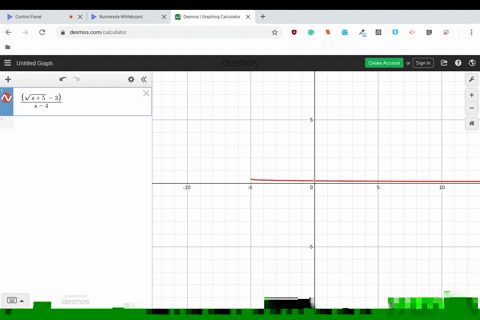Enroll in one of our FREE online STEM bootcamps. Join today and start acing your classes!View Bootcamps02:51
Problem 58

# Finding a Limit What is the limit of $g(x)=x$ as $x$ approaches $\pi ?$

## Discussion

You must be signed in to discuss.

## Video Transcript

in this problem who want to show that the limit is X approaches. Pi of the function f of X is equal to pi. Now we know that the limit is pie because if we look at the graph of F which is depicted here on the left, we can see that after Lex approaches pi from the left hand side of X equals pi Analects approaches pi as well on the right side of X equals pi. So from the graph, we can conclude that the limit is part. Now we want to prove pious the limit by using the Epsilon Delta definition off limits. That is, we want to show that for every excellent greater than zero, there's a delta greater than zero such that X minus pi. Absolute Valium is less than epsilon whenever the absolute value of X minus pi is in between zero and delta. So here we let Delta Echo Absalon. By doing that, we see that the absolute value of X minus pine is then less than delta which is equal to Absalon. So consequently the absolute value of X minus pi is less than excellent and we're done No